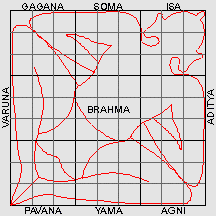# Vaastu: Deformed Plot 1Vaastu Sastra
CHAPTER 14
VAASTU ANALYSIS OF DEFORMED PLOTS – I
Let us in this article start discussion on all possible deformation of shapes of plots (sites). In practice, it is very rare to find a plot that is regular in shape like a rectangle or square, and also that is properly aligned to the magnetic directions.
Majority of plots will have one or both of the following deformations:-
a) axial deformation
b) actual shape deformation
Axial deformation: A plot is said to be axially deformed when its shape is a perfect rectangle but its sides arel not parallel to the directional axis (Fig-1). In Vaastu, we expect the side of the plot to be parallel to the axis of the magnetic directions. Whenever there is an axial tilt towards any magnetic direction, it will cause expansion /extension or retraction /reduction in specific magnetic direction. These aspects have to be analyzed and corrections are to be effected so that as per Vaastu, the plot is made effective.
Actual deformation: In some plots, one or more sides would have extended or retracted from the shape of the rectangle (Fig-2). Also some sides of the plot will be parallel to the magnetic axis. In some other cases, one specific side of the plot might cause deformation by bending outward or inward. In certain plots, a small portion might extend out of the plot, or a small chunk might have got cut from the plot. Also in some cases, the corners of the plots can be truncated or rounded thereby deforming the shape.
All the above will lead to shape deformation of the plot and in each case, correction is to be effected so that the plot becomes suitable for implementing the rules of Vaastu Shastra. In essence, the deformation of a plot leads to one or more of the following results:-
a) Expansion of the plot in a particular direction
b) Extension of the plot towards a particular direction
c) Angular retraction
d) Retraction as square
e) Chopping of one or more corners
f) Curving of one or more corners
g) A diagonal plot
h) A semi-diagonal plot
Let us first discuss about the expansion of plots. The angular growth of a plot towards one magnetic direction is termed as expansion. In expanded plots, the angle of one corner of the expanded side will be much more than 90� while the angle of the other corner of the expanded side will be much less than 90�. The expansion phenomenon can be beneficial in certain directions and detrimental in others. There can be eight such expansions possible in a plot.
In the next article, we will discuss about Vaastu effects caused by deformation due to extension in the plots.
This research article is submitted by Guruji Dr. K. Venkatesan, BE, MTech, EdD, PhD, DDiv